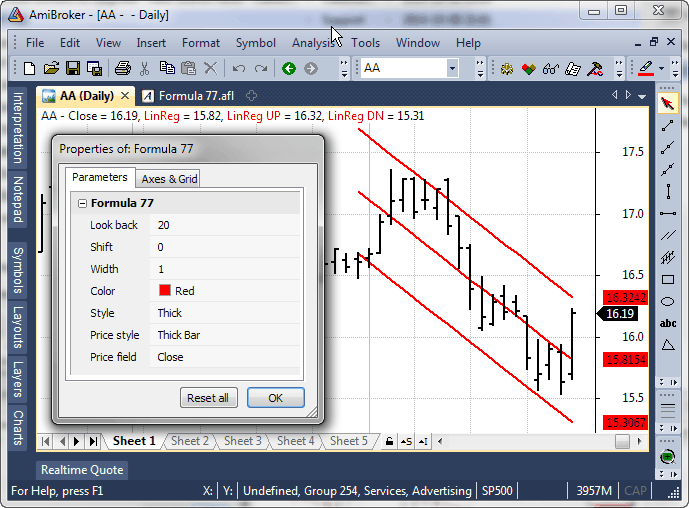#amibroker

### How to draw regression channel programatically

Built-in drawing tool allows to place regression channel on the chart manually and the study works on regular Close array as input. The power of AFL allows to automate this task and draw a customizable regression channel automatically in the chart or choose any custom array for calculation.

Here is a sample coding solution showing how to code Standard Deviation based channel. The Parameters dialog allows to control the array the channel is based upon, number of periods used for calculation, position and width of the channel.

`lookback = Param( "Look back", 20, 1, 200, 1 );shift = Param( "Shift", 0, 0, 20, 1 );multiplier = Param( "Width", 1, 0.25, 5, 0.25 );color = ParamColor( "Color", colorRed );style = ParamStyle( "Style", styleLine | styleDots );pricestyle = ParamStyle( "Price style", styleBar | styleThick, maskPrice );//// price chartPlot( Close, "Close", colorDefault, pricestyle );//array = ParamField( "Price field", -1 );//x = BarIndex() + 1;lastx = LastValue( x ) - shift;//// compute linear regression coefficientsaa = LastValue( Ref( LinRegIntercept( array, lookback ), -shift ) );bb = LastValue( Ref( LinRegSlope( array, lookback ), -shift ) );//// the equation for straight liney = Aa + bb * ( x - ( Lastx - lookback + 1 ) );//width = LastValue( Ref( StDev( array, lookback ), -shift ) );//drawit = x > ( lastx - lookback ) AND BarIndex() < Lastx;//// draw regression line...Plot( IIf( drawit, y, Null ), "LinReg", color, style );// ... and channelPlot( IIf( drawit, y + width*multiplier , Null ), "LinReg UP", color, style );Plot( IIf( drawit, y - width*multiplier , Null ), "LinReg DN", color, style )`

Here is the picture that shows how it looks: